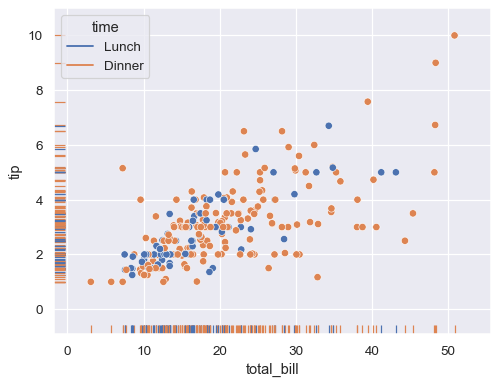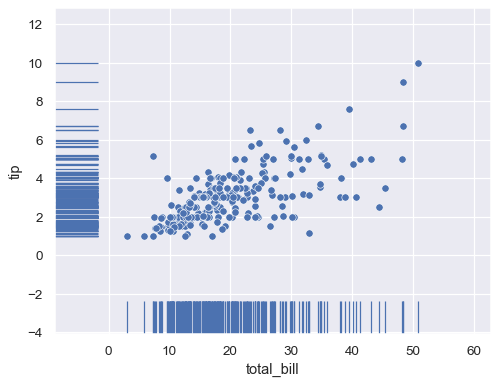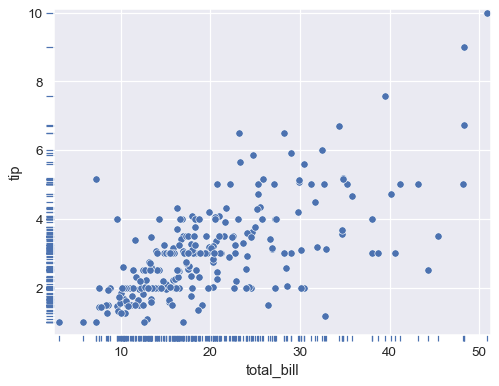# seaborn.rugplot#

seaborn.rugplot(data=None, *, x=None, y=None, hue=None, height=0.025, expand_margins=True, palette=None, hue_order=None, hue_norm=None, legend=True, ax=None, **kwargs)#

Plot marginal distributions by drawing ticks along the x and y axes.

This function is intended to complement other plots by showing the location of individual observations in an unobtrusive way.

Parameters:
data`pandas.DataFrame`, `numpy.ndarray`, mapping, or sequence

Input data structure. Either a long-form collection of vectors that can be assigned to named variables or a wide-form dataset that will be internally reshaped.

x, yvectors or keys in `data`

Variables that specify positions on the x and y axes.

huevector or key in `data`

Semantic variable that is mapped to determine the color of plot elements.

heightfloat

Proportion of axes extent covered by each rug element. Can be negative.

expand_marginsbool

If True, increase the axes margins by the height of the rug to avoid overlap with other elements.

palettestring, list, dict, or `matplotlib.colors.Colormap`

Method for choosing the colors to use when mapping the `hue` semantic. String values are passed to `color_palette()`. List or dict values imply categorical mapping, while a colormap object implies numeric mapping.

hue_ordervector of strings

Specify the order of processing and plotting for categorical levels of the `hue` semantic.

hue_norm

Either a pair of values that set the normalization range in data units or an object that will map from data units into a [0, 1] interval. Usage implies numeric mapping.

legendbool

If False, do not add a legend for semantic variables.

ax`matplotlib.axes.Axes`

Pre-existing axes for the plot. Otherwise, call `matplotlib.pyplot.gca()` internally.

kwargs

Other keyword arguments are passed to `matplotlib.collections.LineCollection()`

Returns:
`matplotlib.axes.Axes`

The matplotlib axes containing the plot.

Examples

Add a rug along one of the axes:

```import seaborn as sns; sns.set_theme()
sns.kdeplot(data=tips, x="total_bill")
sns.rugplot(data=tips, x="total_bill")
```Add a rug along both axes:

```sns.scatterplot(data=tips, x="total_bill", y="tip")
sns.rugplot(data=tips, x="total_bill", y="tip")
```Represent a third variable with hue mapping:

```sns.scatterplot(data=tips, x="total_bill", y="tip", hue="time")
sns.rugplot(data=tips, x="total_bill", y="tip", hue="time")
```Draw a taller rug:

```sns.scatterplot(data=tips, x="total_bill", y="tip")
sns.rugplot(data=tips, x="total_bill", y="tip", height=.1)
```Put the rug outside the axes:

```sns.scatterplot(data=tips, x="total_bill", y="tip")
sns.rugplot(data=tips, x="total_bill", y="tip", height=-.02, clip_on=False)
```Show the density of a larger dataset using thinner lines and alpha blending:

```diamonds = sns.load_dataset("diamonds")
sns.scatterplot(data=diamonds, x="carat", y="price", s=5)
sns.rugplot(data=diamonds, x="carat", y="price", lw=1, alpha=.005)
```